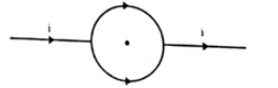# A conducting circular loop of radius a is connected to two long,

Question:

A conducting circular loop of radius a is connected to two long, straight wires. The straight wires carry current $i$ as shown in figure. Find the magnetic field $B$ at the center of the loop.Solution:

Current in each semi-circle $=\frac{\mathrm{i}}{2}$

Magneti field due to each semicircle is same but in opposite direction. So, net magnetic field is zero.